Right-Brained Fractions

# Right-Brained Fractions

Fractions are difficult for many students to learn, but Right-Brained Fractions can help! Here are a few reasons why children struggle:

##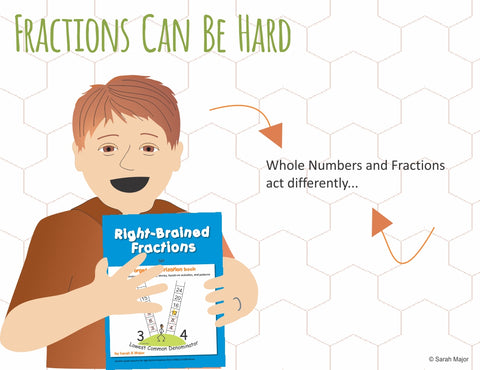By the time children tackle fractions, they have an understanding of whole numbers, and fractions don’t behave like whole numbers do.In whole numbers, the larger the number, the larger the amount (4 is larger than 2), while in fractions, the larger the denominator, the smaller the amount will be.You cannot count fractions like you can count whole numbers. Between each fraction is an infinite number of possible fractions.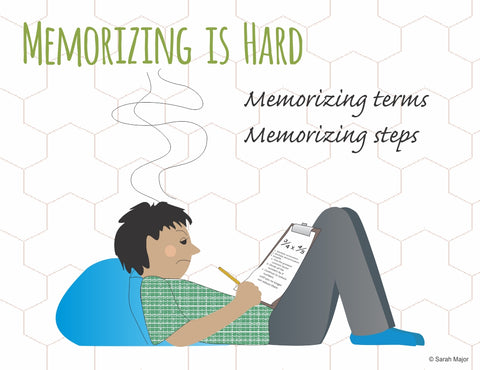Fractions are taught relying heavily on memorizing many terms and procedural steps. For those children who cannot learn via memorization and don’t learn using steps in a procedure, learning how to compute with fractions is nearly impossible.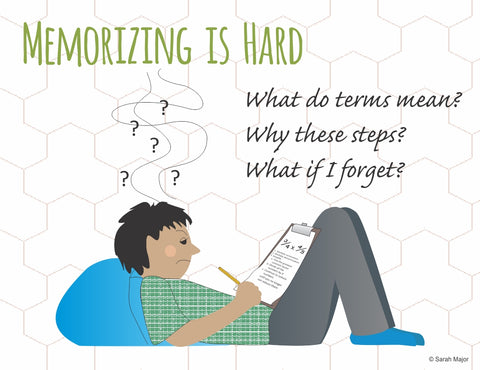Fractions and their associated rules are pretty much meaningless to students. They don’t understand what is happening in each step so the steps are hard to remember.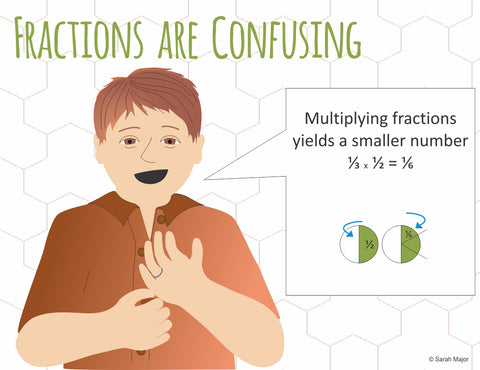Multiplication and division of fractions acts exactly opposite to the same processes with whole numbers. For example, with whole numbers, multiplying results in a larger number. With fractions, multiplying results in a smaller number.

## How Right-Brained Fractions addresses these difficulties:Every term is defined in such a way that the very structure of each word is explored for its meaning. For example, “Numerator” is broken into two parts: Ator means “one who” and Numer means “enumerates or counts” which means that the numerator is one who counts.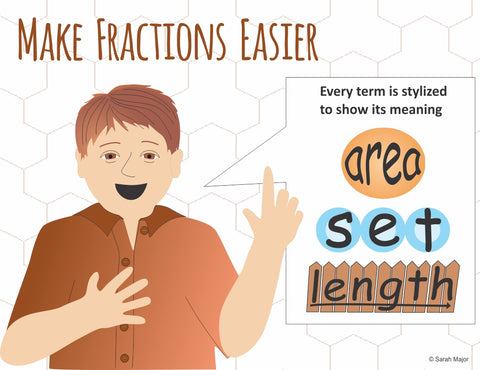Each term is also stylized in full color to show the meaning of the word.Real life stories introduce students to the meaning behind the various steps in fractions which help students remember what to do and why they are doing it. (Thus avoiding the need for memorization of steps).Every lesson has hands-on components before the student ever deals with symbols and puts pencil to paper to solve problems.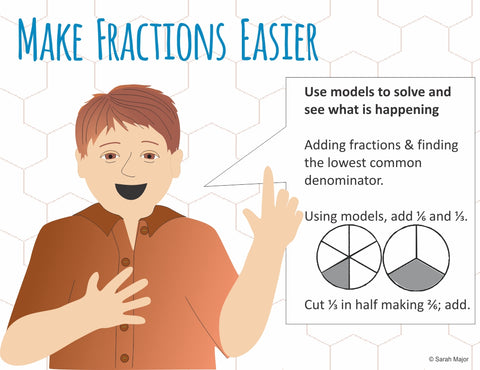Models are used consistently so that the student can see what is happening rather than just listening to an explanation of what is happening.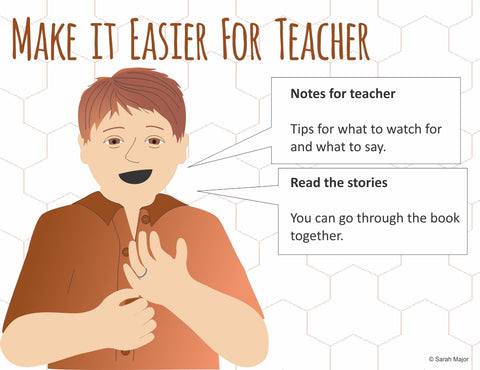Notes to the teacher as well as stories to read make teaching fractions simple for the teacher without having to engage in extensive training ahead of time. You can actually read through the book with your students.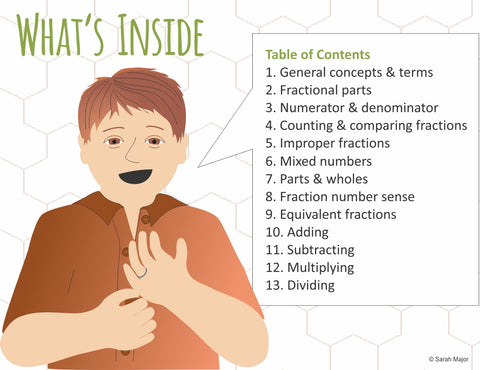All activities, worksheets, assessments, and answer keys are included. The book is ready to pick up and use.

Right-Brained Fractions is broken into 14 chapters which begin with General Concepts and terms and end with dividing fractions.

Pick up a copy of Right-Brained Fractions today and give your visual child the solution to their fraction problems.